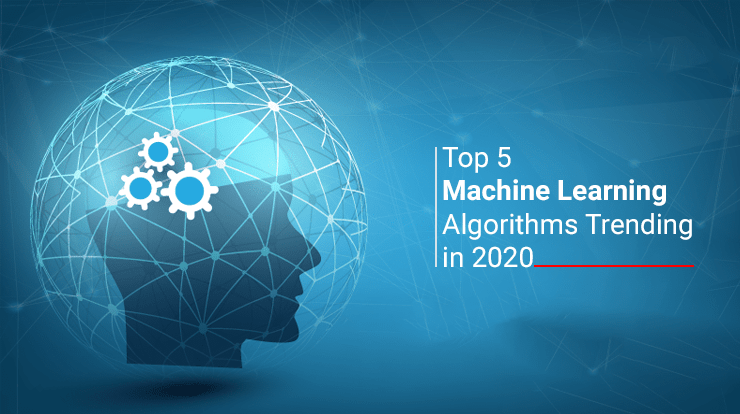# Top 5 Machine Learning Algorithms Trending In 2020This machine learning algorithm is based on supervised learning and revolves around the input and output variables using an algorithm to predict the outcome. It runs a regression task. It models a target prediction value based on independent variables, which is mostly used for finding out the relationship between variables & forecasting.

In this technique, a relationship is established among independent and dependent variables by bringing them to a line. This line is known as the regression line and expressed by a linear equation Y= a*X + b.

Where:

Y = Dependent Variable

a = Slope

X = Independent variable

b = Intercept

We get coefficients ‘a’ & ‘b’ by minimizing the sum of the squared difference of distance between data points and the regression line.

Logical regression:

Identical to linear regression, logical regression is another statistical method for classification which finds the values for two coefficients that weigh each input variable. The difference between the two is that, this solves problems of binary classification, relying on a logical, non-linear function instead. Hence, logical regression determines whether a data instance belongs to one class or another and can also provide the reasoning behind the prediction, unlike linear regression.

When using this algorithm, limiting correlating data and eliminating noise is also necessary.

Classification and Regression Trees:

Decision Trees are a significant type of algorithm for predictive modeling machine learning.

These decision tree algorithms have been around for decades and modern variations like random forest are among the most robust techniques available. Classification and Regression Trees or ‘CART’ is a term introduced by a distinguished statistician Leo Breiman to refer to Decision Tree algorithms that can be used for classification or regression predictive modeling problems. Generally, it is referred to as “decision trees”, but on few platforms like R they are referred by the more modern term CART.

In this algorithm, we divide the population into two or more homogeneous sets based on the most relevant attributes/ independent variables.

K-nearest neighbor (KNN):

KNN is an acronym for the K-nearest neighbor method, in which the user defines the value of K. Unlike previous algorithms, this one trains on the entire data-set.

The algorithm guides the machine to check the entire data-set to find the k-nearest instances to this new data instance or to find the k-number of instances that are the most closely related to the new instance. The prediction or output can be one of the below two things:

– The mode of most frequent class, in a classification problem

– The mean of the outcomes, in a regression problem

This algorithm can be easily understood by comparing it with real life situations, likewise if you want to know about a person, you should ask about him/ her from his friends or colleagues.

Naïve Bayes:

To calculate the probability that if an event will occur or not, given that another event has already occurred, we use Bayes’s Theorem, which is: P(h|d)= (P(d|h) P(h)) / P(d)

In this equation:

P(h|d) = Posterior probability i.e. the probability of hypothesis h being true, given the data d, where P(h|d)= P(d1| h) P(d2| h)….P(dn| h) P(d)

P(d|h) = Likelihood i.e. the probability of data d given that the hypothesis h was true.

P(h) = Class prior probability i.e. the probability of hypothesis h being true (irrespective of the data)

P(d) = Predictor prior probability i.e. probability of the data (irrespective of the hypothesis)

This algorithm is known as ‘naive’ because it assumes that all the variables are independent of each other, which is a naive assumption to make in real-world examples.

Conclusion:

Applying these five machine learning algorithms may not be too complicated, but they do take time to master. These are some important building blocks that can serve as a solid starting point for further study of more advanced algorithms and methods.